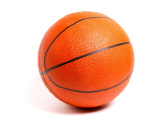Name:    1MD1

Multiple Choice
Identify the choice that best completes the statement or answers the question.

1.

Which of the following objects is the shortest in length?
 a.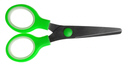b.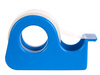c.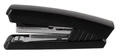2.

Which of these items is about the length of 3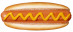?
 a.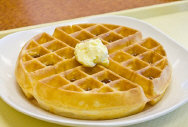b.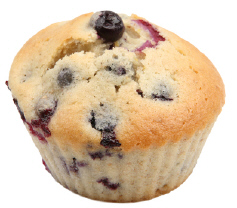3.

Which object is about the same size as one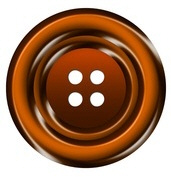?
 a.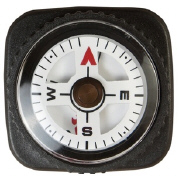b.4.

Which of these pictures is about two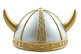long?
 a.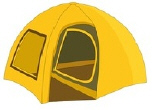b.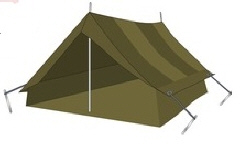5.

Which of these objects is about three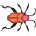long?
 a.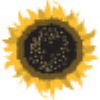b.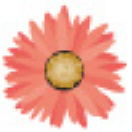6.

Which of the following objects is the widest?
 a.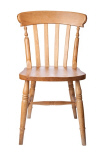b.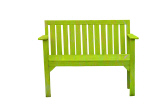c.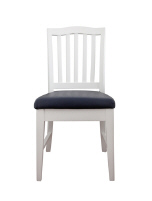7.

Which of the following objects is the shortest in height?
 a.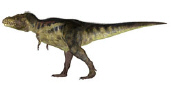b.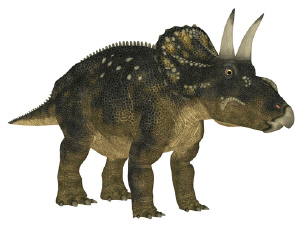c.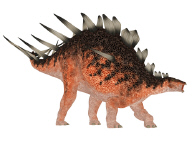8.

Which of the following objects is the tallest in height?
 a.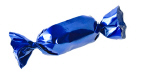b.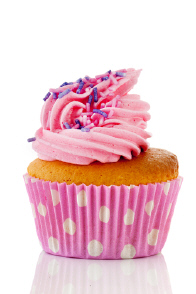c.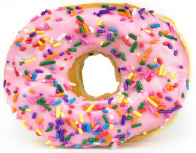9.

Which of the following objects is the shortest in height?
 a.b.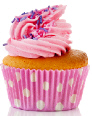c.10.

Which of these items is about the length of 2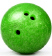a.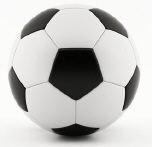b.